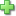# Life of Fred: Complex Analysis

by Stanley F. Schmidt
Hardcover, 176 pages
Price: \$49.00

Complex Analysis is the theory of functions of a single complex variable.  (That is: Functions that map from complex numbers to complex numbers.)  This is advanced undergraduate mathematics with applications to even more advanced mathematics, physics, and engineering.  Some of the topics covered:

• Fred's scheduling issues
• Cauchy-Riemann equations; Cauchy-Goursat theorem; Cauchy’s formula
• Derivatives and Integration in the set of complex numbers
• Casorati-Weierstrass theorem; Picard’s theorems; Liouville theorem
• Why the Residue theorem is called a residue
• Red Riding Hood

Topics Covered:

Arithmetic in the complex plane. Conjugates and absolute value. Polar form. Complex functions. Graphing. Limits and derivatives. Cauchy-Riemann equations. Holomorphic functions. Regions, open sets, connected sets. Paths. Analytic functions. Integration along a path. Fundamental Theorem of Complex Integration. Piecewise smooth paths. Paths with corners or cusps. Cauchy’s theorem. Closed paths. Simple paths. Cauchy–Goursat theorem. Trig functions, exponents, and logs in the complex plane. Argument of a polar complex function. Morera’s theorem. Three kinds of isolated singular points. Order of a pole. Proving L’Hospital’s rule in the complex plane. Meromorphic functions. Casorati-Weierstrass theorem. Picard’s great theorem. Cauchy’s formula. Jordan curve theorem. Liouville theorem. Proof of the Fundamental Theorem of Algebra. Taylor series and Laurent series. Residue theorem. Analytic continuation. Riemann Zeta function. Conformal Mappings. Homotopic paths. Harmonic functions.

Did you find this review helpful?
Series Description
Recommended for...
Customer ReviewsClick here to write a review